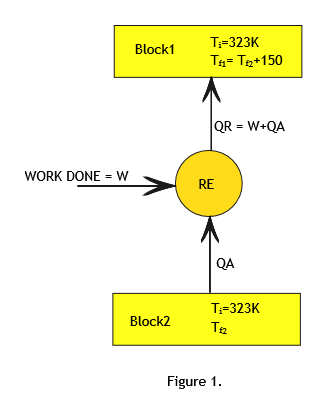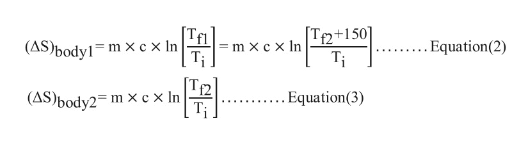# Two identical metal blocks of mass 20 kg and specific heat 0.4 kJ/kg are at an initial temperature of 50 degree Celsius .A reversible refrigerator receives heat from one block and rejects heat to the other. Find the work required by the refrigerator to cause a temperature difference of 150 degree Celsius between the two blocks.

Question

Two identical metal blocks of mass 20 kg and specific heat 0.4 kJ/kg are at an initial temperature of 50 degree Celsius .A reversible refrigerator receives heat from one block and rejects heat to the other. Find the work required by the refrigerator to cause a temperature difference of 150 degree Celsius between the two blocks.

## Expert Answer

19 Ratings
Step 1

Let us call the two blocks as block1 and block2. (Refer to Figure1)

Mass of each block (m)= 20kg.

Specific heat of each block (c)=0.4 kJ/kg.

Initial temperature of both the blocks is same (Ti)= 500C=323K.

Final Temperature of block1=Tf1.

Final Temperature of block2=Tf2.

Since heat is absorbed from block2 and  rejected to block1 ,Tf1 > Tf2  and it is given that Tf1 - Tf2 = 150.fullscreen
Step 2

Since the refrigerator is reversible refrigerator all the processes involved are reversible processes.
According to Principle of entropy, for reversible processes change in entropy of the Universe is zero.fullscreen
Step 3

Calculate change in entropy of body1 and body2 ...fullscreen

### Ask a Homework Question!

Subscribe to bartleby learn and ask 30 homework questions each month. Subject matter experts are on standby 24/7 when you’re stuck and questions are typically answered in as fast as 30 minutes.*

*Response times may vary by subject and question complexity. Median response time is 34 minutes for paid subscribers and may be longer for promotional offers.

### Ask a Homework Question!

Subscribe to bartleby learn and ask 30 homework questions each month. Subject matter experts are on standby 24/7 when you’re stuck and questions are typically answered in as fast as 30 minutes.*

Ask a Question

*Response times may vary by subject and question complexity. Median response time is 34 minutes for paid subscribers and may be longer for promotional offers.

Tagged in
Engineering
Mechanical Engineering

### Thermodynamics© 2021 bartleby. All Rights Reserved.